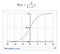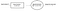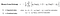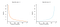# Logistic Regression — A supervised Learning (Classification) Algorithm

We already covered one of the regression algorithms (i.e) Linear Regression(Day6). Now, We can switch over to the next type of supervised learning which is Classification. The output target is a categorical variable such as (Human or Not Human), (Good, Neutral or bad) or (Advanced, Intermediate or Novice). The must-know algorithm for the classification type problem is Logistic Regression.

Table of contents:

1. What is a sigmoid function?

2. Forward Propagation in Logistic Regression

3. Binary Cross-Entropy — A loss function

4. Backward Propagation for Logistic Regression

5. One Vs rest

What is a sigmoid function: In the linear regression, we used a straight line equation y=mx+c to find the relation between input(x) and numerical output(y). Having said that, the logistic regression aims to create categorical results ‘0’ or ‘1’ with the help of sigmoid function S(x) = 1 / (1+e^-x). when

x=0 , S(x) = 0.5

x > 0, S(x) = slowly increasing from 0.5 then flattens out at 1

x <0, S(x) = slowly decreasing from 0.5 then levels out at 0Fig1 — shows sigmoid function from wiki

In machine learning, the sigmoid function is termed as an activation function. The characteristics of the sigmoid include non-linearity between input and target, the output value is compressed between 0 &1 and monotonic in nature(follows one single direction either increasing or decreasing).

Forward Propagation in Logistic Regression — During the forward pass, the dependent target is determined by first calculating the linear function followed by the sigmoid activation.Fig2 — shows forward pass of logistic regression

A threshold is set (usually 0.5), any value falls above the limit will be treated as ‘1’ or ‘0’ otherwise. We can imagine the sigmoid activation function is like a neuron in the brain (an on or off switch). This forms the basis of neural networks that will be covered later.

We may wonder, why the name ‘logistic regression’ when we are actually dealing with a classification output. There is definitely a mathematical justification for that. Let’s closely scrutinize the sigmoid function itself.

y(z) = 1 / (1 + e^-z)

Taking logarithm on both sides,

log(y(z)) = log(1 / (1 + e^-z))

Applying logarithmic properties(division rule),

log(y(z)) = log(1) — log(1 + e^-z)

Applying logarithmic properties(addition rule),

log(y(z)) = log(1) — log(1) — log(e^-z)

log(1) = 0 and log(e^-z) = -z

log(y(z)) = z

we know z = w*x + b

log(y(z)) = w*x + b . This equation resembles like a linear regression except the left side has a log function in it. For the very same reason, it is termed as ‘logistic regression’.

Binary Cross-Entropy (log-likelihood) — A loss function: For classification, the mean_squared_error used for regression would not be applicable. As we are concerned about the categorical output rather than numerical value, the apt function is Binary Cross Entropy. Thinking back to one of the salient properties of the loss function (i.e) Covex in nature, making it easier to take gradient descent towards the global minima. At the same time, the function should be effective enough to assess the accuracy of the output labels.The formula for Binary Cross-EntropyFig 3 — shows log loss curves

In the above figures, when the predicted probability and the expected value are the same, then the loss value is minimal else there will be a high penalty resulting in huge loss value.

Using the gradient descent algorithm the parameters are updated recursively, till an acceptable cost is attained.

Backward Propagation for Logistic Regression — By taking the derivative with respect to the learnable parameters ‘weight’ and ‘bias’, the log error is minimized in each iteration. In the below J refers to the binary cross-entropy cost function.The derivative of loss function w.r.t weights and bias

m — refers to the number of training samples.

Update the weights and bias using the derivative values obtained above as well as with the help of the learning rate.

α — learning rate which is a constant real number(same meaning used for linear regression)

Weights = Weights — α * derivative of the loss function with respect to W

bias = bias — α * derivative of the loss function with respect to b

One Vs rest — Since logistic regression outputs two labels, it best fits for binary classification problem. But if we want to leverage the algorithm for multilabel classification, then it could be implemented through One Vs rest / One Vs all.

Consider the scenario of movie reviews (Good, Bad or Neutral).

Classifier 1: Good Vs (Bad & Neutral), Classifier 2: Bad Vs (Good & Neutral), Classifier 3: Neutral Vs (Good & Bad)

The labels in the brackets will be given a single name and every classifier mentioned above will act as a binary classifier. Finally, whichever classifier gives the highest predicted probability, it would be taken forward. The constraint with this method is enormous computation cost, as the data has to go through all the classifiers to decide the probability.

Recommended Reading:

AI Enthusiast | Blogger✍

## More from Nandhini N

AI Enthusiast | Blogger✍